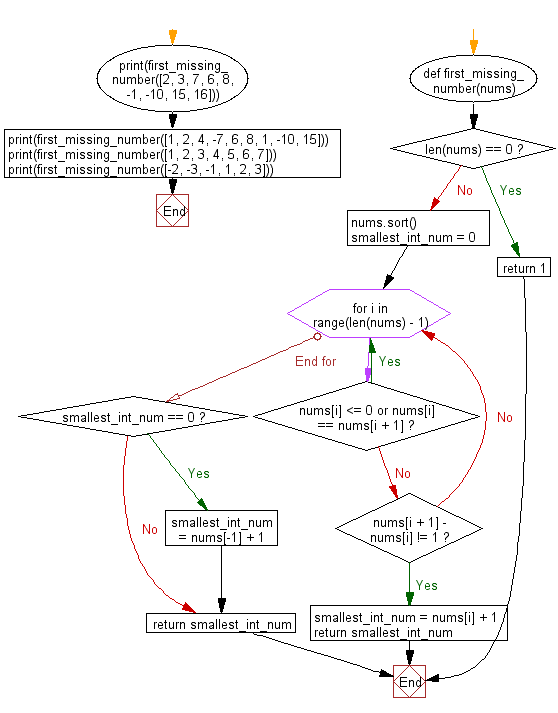﻿ Python: Find the first missing positive integer that does not exist in a given list - w3resource# Python: Find the first missing positive integer that does not exist in a given list

## Python Basic - 1: Exercise-80 with Solution

Write a Python program to find the first missing positive integer that does not exist in a given list.

Sample Solution:

Python Code:

``````def first_missing_number(nums):
if len(nums) == 0:
return 1

nums.sort()
smallest_int_num = 0

for i in range(len(nums) - 1):

if nums[i] <= 0 or nums[i] == nums[i + 1]:
continue
else:
if nums[i + 1] - nums[i] != 1:
smallest_int_num = nums[i] + 1
return smallest_int_num
if smallest_int_num == 0:
smallest_int_num = nums[-1] + 1
return smallest_int_num

print(first_missing_number([2, 3, 7, 6, 8, -1, -10, 15, 16]))
print(first_missing_number([1, 2, 4, -7, 6, 8, 1, -10, 15]))
print(first_missing_number([1, 2, 3, 4, 5, 6, 7]))
print(first_missing_number([-2, -3, -1, 1, 2, 3]))
``````

Sample Output:

```4
3
8
4
```

Flowchart:Python Code Editor:

Have another way to solve this solution? Contribute your code (and comments) through Disqus.

What is the difficulty level of this exercise?

Test your Programming skills with w3resource's quiz.

﻿

## Python: Tips of the Day

How to make a flat list out of list of lists?

Given a list of lists l

```flat_list = [item for sublist in l for item in sublist]
```

which means:

```flat_list = []
for sublist in l:
for item in sublist:
flat_list.append(item)
```

is faster than the shortcuts posted so far. (l is the list to flatten.) Here is the corresponding function:

flatten = lambda l: [item for sublist in l for item in sublist]

As evidence, you can use the timeit module in the standard library:

```\$ python -mtimeit -s'l=[[1,2,3],[4,5,6], , [8,9]]*99' '[item for sublist in l for item in sublist]'
10000 loops, best of 3: 143 usec per loop
\$ python -mtimeit -s'l=[[1,2,3],[4,5,6], , [8,9]]*99' 'sum(l, [])'
1000 loops, best of 3: 969 usec per loop
\$ python -mtimeit -s'l=[[1,2,3],[4,5,6], , [8,9]]*99' 'reduce(lambda x,y: x+y,l)'
1000 loops, best of 3: 1.1 msec per loop
```

Explanation: the shortcuts based on + (including the implied use in sum) are, of necessity, O(L**2) when there are L sublists -- as the intermediate result list keeps getting longer, at each step a new intermediate result list object gets allocated, and all the items in the previous intermediate result must be copied over (as well as a few new ones added at the end). So, for simplicity and without actual loss of generality, say you have L sublists of I items each: the first I items are copied back and forth L-1 times, the second I items L-2 times, and so on; total number of copies is I times the sum of x for x from 1 to L excluded, i.e., I * (L**2)/2.

The list comprehension just generates one list, once, and copies each item over (from its original place of residence to the result list) also exactly once.

Ref: https://bit.ly/3dKsNTR